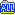# C# と VB.NET の質問掲示板

## ASP.NET、C++/CLI、Java 何でもどうぞ

### C# と VB.NET の入門サイト

#### 三目並べ　勝敗　配列？

[トピック内 4 記事 (1 - 4 表示)]　 << 0 >>

 ■92651 / inTopicNo.1) 三目並べ　勝敗　配列？
□投稿者/ aiti (1回)-(2019/10/17(Thu) 17:35:39)
 分類:[VB.NET/VB2005 以降]　 '勝敗判定 If table(0, 0) = "O" And table(0, 1) = "O" And table(0, 2) = "O" Then Gameover = 1 WinPlayer = P1name ElseIf table(1, 0) = "O" And table(1, 1) = "O" And table(1, 2) = "O" Then Gameover = 1 WinPlayer = P1name ElseIf table(2, 0) = "O" And table(2, 1) = "O" And table(2, 2) = "O" Then Gameover = 1 WinPlayer = P1name ElseIf table(0, 0) = "O" And table(1, 0) = "O" And table(2, 0) = "O" Then Gameover = 1 WinPlayer = P1name ElseIf table(0, 1) = "O" And table(1, 1) = "O" And table(2, 1) = "O" Then Gameover = 1 WinPlayer = P1name ElseIf table(0, 2) = "O" And table(1, 2) = "O" And table(2, 2) = "O" Then Gameover = 1 WinPlayer = P1name ElseIf table(0, 0) = "O" And table(1, 1) = "O" And table(2, 2) = "O" Then Gameover = 1 WinPlayer = P1name ElseIf table(0, 2) = "O" And table(1, 1) = "O" And table(2, 0) = "O" Then Gameover = 1 WinPlayer = P1name ElseIf table(0, 0) = "X" And table(0, 1) = "X" And table(0, 2) = "X" Then Gameover = 1 WinPlayer = P2name ElseIf table(1, 0) = "X" And table(1, 1) = "X" And table(1, 2) = "X" Then Gameover = 1 WinPlayer = P2name ElseIf table(2, 0) = "X" And table(2, 1) = "X" And table(2, 2) = "X" Then Gameover = 1 WinPlayer = P2name ElseIf table(0, 0) = "X" And table(1, 0) = "X" And table(2, 0) = "X" Then Gameover = 1 WinPlayer = P2name ElseIf table(0, 1) = "X" And table(1, 1) = "X" And table(2, 1) = "X" Then Gameover = 1 WinPlayer = P2name ElseIf table(0, 2) = "X" And table(1, 2) = "X" And table(2, 2) = "X" Then Gameover = 1 WinPlayer = P2name ElseIf table(0, 0) = "X" And table(1, 1) = "X" And table(2, 2) = "X" Then Gameover = 1 WinPlayer = P2name ElseIf table(0, 2) = "X" And table(1, 1) = "X" And table(2, 0) = "X" Then Gameover = 1 WinPlayer = P2name ElseIf LoopCount1 > 9 Then Gameover = 2 End Ifとりあえず、勝敗の全パターンを書きましたが、いまいち配列化？関数化のやり方がわかりません。

 ■92652 / inTopicNo.2) Re: 三目並べ　勝敗　配列？
□投稿者/ キングダム (46回)-(2019/10/17(Thu) 19:56:12)
 ループを使えばなにかできそうな気がします処理をわけるなら・横に並んでる・縦に並んでる・斜めに並んでるをチェックする処理にするかなーと思いました分けるかは悩みどころですけど

 ■92653 / inTopicNo.3) Re: 三目並べ　勝敗　配列？
□投稿者/ 魔界の仮面弁士 (2426回)-(2019/10/17(Thu) 21:26:11)
 ■No92651 (aiti さん) に返信> '勝敗判定And ではなく、AndAlso を使うべきかと。> 勝敗の全パターンを書きましたが、「まだ勝敗が付いていない段階」の値が未定義に見えます。Else　Gameover = 0　WinPlayer = NothingEnd If> いまいち配列化？関数化のやり方がわかりません。いろいろな方法があるとは思いますが、一例として。'縦・横・斜めの探索ライン(8通り)Dim lines(,) As Integer = {　{0, 3, 6}, {1, 4, 7}, {2, 5, 8},　{0, 1, 2}, {3, 4, 5}, {6, 7, 8},　{0, 4, 8}, {2, 4, 6}}'勝敗が決まっていない時の初期値Gameover = 0WinPlayer = Nothing'全パターン 8 回探索するFor n = 0 To 7　Dim v() As Integer = {lines(n, 0), lines(n, 1), lines(n, 2)}　Dim line = String.Join("", v.Select(Function(p) table(p Mod 3, p \ 3)))　' 3 つ揃ったラインがあればループ終了　If line = "OOO" Then　　Gameover = 1　　WinPlayer = P1name　　Exit For　ElseIf line = "XXX" Then　　Gameover = 2　　WinPlayer = P2name　　Exit For　End IfNext

 ■92663 / inTopicNo.4) Re: 三目並べ　勝敗　配列？
□投稿者/ aiti (4回)-(2019/10/18(Fri) 15:25:48)
 ■No92653 (魔界の仮面弁士 さん) に返信■No92651 (キングダム さん) に返信お二方回答ありがとうございました。まだVBを使い始めたばかりなので、あれですが、かなりの行の短縮、まとめることができるということを知りました。このトピックをツリーで一括表示

トピック内ページ移動 / << 0 >>

このトピックに書きこむ
• 入力内容にタグは利用できません。
• 他人を中傷する記事は管理者の判断で予告無く削除されます。
• 半角カナは使用しないでください。文字化けの原因になります。
• 名前、コメントは必須記入項目です。記入漏れはエラーになります。
• 入力内容の一部は、次回投稿時の手間を省くためブラウザに記録されます。
• URL は自動的にリンクされます。
• 記事中に No*** のように書くとその記事にリンクされます(No は半角英字/*** は半角数字)。

 名前 (*必須) E-Mail (任意) Web サイト (任意) 投稿モード 通常モード　　図表モード (ソースコードを貼る場合はこちらを使用) 本文　(質問の場合は、開発環境・使用言語のバージョンなどを最初に書いてください) ■No92663 (aiti さん) に返信 > ■No92653 (魔界の仮面弁士 さん) に返信 > ■No92651 (キングダム さん) に返信 > お二方回答ありがとうございました。まだVBを使い始めたばかりなので、あれですが、かなりの行の短縮、まとめることができるということを知りました。 編集キー 自分の投稿を編集する時に必要です (半角8文字以内) 解決済み /解決したらチェックしておきましょう。 プレビュー/

管理者用